# In an experiment, 24.5 g of metal was heated to 98.0°C and then quickly transferred to...

In an experiment, 24.5 g of metal was heated to 98.0°C and then quickly transferred to 150.0 g of water in a calorimeter. The initial temperature of the water was 27.0°C, and the final temperature after the addition of the metal was 32.5°C. Assume the calorimeter behaves ideally and does not absorb or release heat.

i keep getting 2.15, but the program is telling me thats wrong

use:
heat released by metal = heat absorbed by solution
24.5*c*(98.0 - 32.5) = 150.0*4.184*(32.5 - 27.0)
1604.75*c = 3451.8
c = 2.15 J/goC

I think you are correct

just focus on unit. In which unit the answer being asked in

#### Earn Coin

Coins can be redeemed for fabulous gifts.

Similar Homework Help Questions
• ### cu 10 Question (1 point In an experiment, 28.5g of metal was heated to 98.0°C and...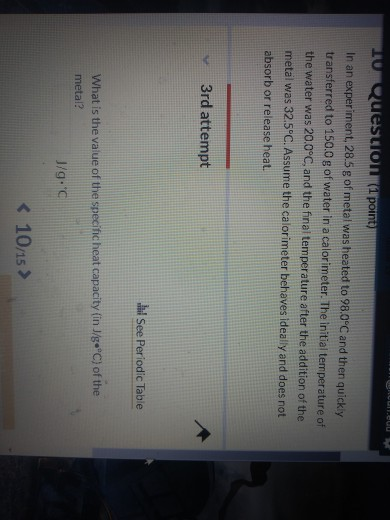cu 10 Question (1 point In an experiment, 28.5g of metal was heated to 98.0°C and then quickly transferred to 150.0 g of water in a calorimeter. The initial temperature of the water was 20.0°C, and the final temperature after the addition of the metal was 32.5°C. Assume the calorimeter behaves ideally and does not absorb or release heat 3rd attempt See Periodic Table What is the value of the specificheat capacity (in J/g °C) of the metal? J/g.c <...

• ### a See page 236 06 Question (1 point) 27.5 g of metal was heated to 98.0°C...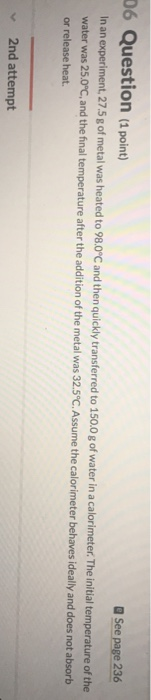a See page 236 06 Question (1 point) 27.5 g of metal was heated to 98.0°C and then quickly transferred to 150.0 g of water in a calorimeter.The initial temperature of the water was 25.0 C.and the final temperature after the addition of the metal was 32.5"C.Assume the calorimeter behaves ideally and does not absorb or release heat. 2nd attempt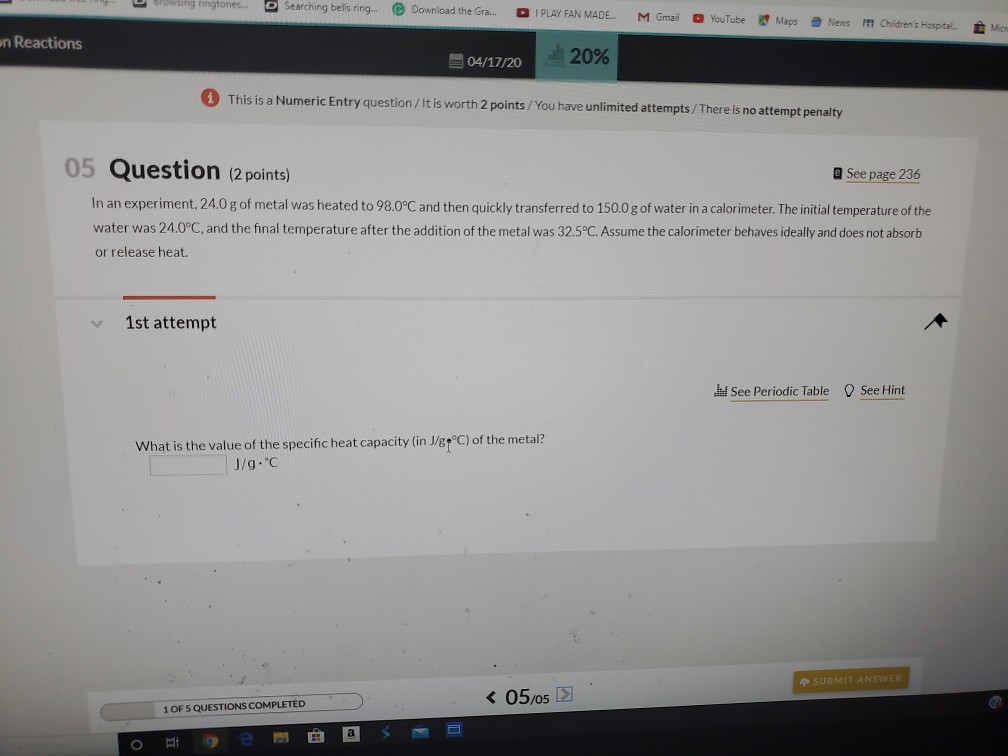- Dowling ringtones. Searching bells ring. Download the Gra... PLAY FAN MADE M Gmail YouTube Maps News Children's Hospital Mic en Reactions 04/17/20 20% 1 This is a Numeric Entry question / It is worth 2 points/ You have unlimited attempts/There is no attempt penalty 05 Question (2 points) @ See page 236 In an experiment, 24.0 g of metal was heated to 98.0°C and then quickly transferred to 150.0 g of water in a calorimeter. The initial temperature of...

• ### A 48.79 g sample of metal is heated to 98.77oC and then quickly transferred to 88.34...

A 48.79 g sample of metal is heated to 98.77oC and then quickly transferred to 88.34 mL of water at 24.65oC contained in a new calorimeter. This calorimeter has a calorimeter constant (heat capacity) with a numerical value of 55.7 (see problem 2e above for appropriate units). The thermal equilibrium temperature of the metal sample plus water mixture was 27.49oC. Assume the density of the water is 1.00 g/mL. a. How much heat in Joules is gained by the water?...

• ### 2. DANS A piece of unknown metal weighs 100.0 g. It is heated to 98.0°C before...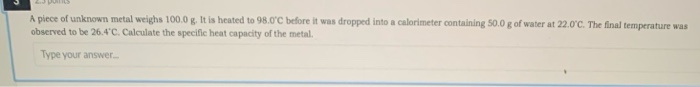2. DANS A piece of unknown metal weighs 100.0 g. It is heated to 98.0°C before it was dropped into a calorimeter containing 50.0 g of water at 22.0°C. The final temperature was observed to be 26.4'C. Calculate the specific heat capacity of the metal. Type your answer

• ### . A 150.0 g sample of a Metal was heated to 95.0°C. When the hot metal...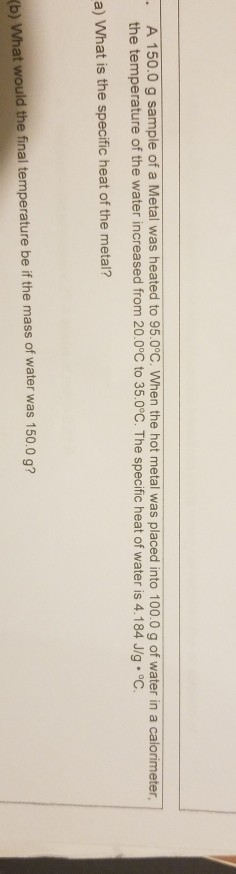. A 150.0 g sample of a Metal was heated to 95.0°C. When the hot metal was placed into 100.0 g of water in a calorimeter, the temperature of the water increased from 20.0°C to 35.0°C. The specific heat of water is 4.184 J/g °C. a) What is the specific heat of the metal? Kb) What would the final temperature be if the mass of water was 150.0 q?

• ### A sample of metal with a mass of 650.0 g is heated to 98.0 degree Celsius...

A sample of metal with a mass of 650.0 g is heated to 98.0 degree Celsius and dropping into 500.0 g of water at 28.4 degree Celsius. The water temperature raises to 39.0 C. Assume there is not heat lost to the environment. Calculate the specific heat of metal.

• ### 4. A 47.4 g sample of a metal is heated to 95.2°C and then placed in...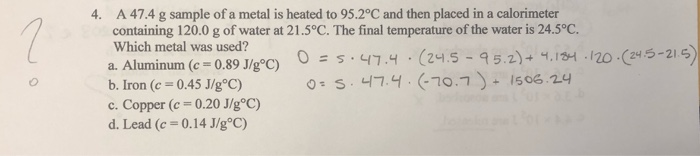4. A 47.4 g sample of a metal is heated to 95.2°C and then placed in a calorimeter containing 120.0 g of water at 21.5°C. The final temperature of the water is 24.5°C. Which metal was used? a. Aluminum (c = 0.89 J/gºC) OES:47.4.(24.5 - 95.2) + 4.184.120 (24.5-215) b. Iron (c = 0.45 J/g °C) o=s.47.4.(-70.7 + 1506.24 c. Copper (c=0.20 J/g°C) d. Lead (c = 0.14 J/g°C)

• ### A metal sample weighing 24.000 g is heated to 100.0 degrees celsius and then transferred into a calorimeter containing 3...

A metal sample weighing 24.000 g is heated to 100.0 degrees celsius and then transferred into a calorimeter containing 30.0 mL of water at a temperature of 22.8 degrees celsius. If the specific heat of the metal is 0.105 J/g*C, what is the final temperature of the metal sample plus water?

• ### A metal object with mass of 24.0 g is heated to 97.0 °C and then transferred...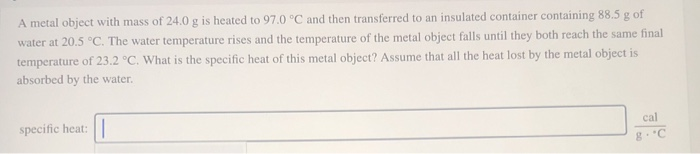A metal object with mass of 24.0 g is heated to 97.0 °C and then transferred to an insulated container containing 88.5 g of water at 20.5 °C. The water temperature rises and the temperature of the metal object falls until they both reach the same final temperature of 23.2 °C. What is the specific heat of this metal object? Assume that all the heat lost by the metal object is absorbed by the water. specific heat: